## Prototype a Component and Get Instant Feedback

This example shows how you can interactively modify the component source and get instant feedback on the resulting block implementation.

To have the block reflect the changes to the underlying source, right-click the block icon and, from the context menu, select Simscape > Refresh source code. If you make a mistake (for example, omit the `end` keyword) when editing the component source, then when you refresh the block, the compiler issues a diagnostic error message, pointing to the appropriate line in the code.

1. Open the Simscape > Foundation Library > Electrical > Electrical Elements > Variable Resistor block dialog box. On the Description tab, click the Source code link. The underlying source code opens in the Editor window.

```component variable_resistor % Variable Resistor :1.5 % Models a linear variable resistor. The relationship between voltage V % and current I is V=I*R where R is the numerical value presented at the % physical signal port R. The Minimum resistance parameter prevents % negative resistance values. % % Connections + and - are conserving electrical ports corresponding to % the positive and negative terminals of the resistor respectively. The % current is positive if it flows from positive to negative, and the % voltage across the resistor is given by V(+)-V(-). % Copyright 2005-2020 The MathWorks, Inc. inputs R = { 0.0, 'Ohm' }; % R:left end nodes p = foundation.electrical.electrical; % +:left n = foundation.electrical.electrical; % -:right end parameters Rmin = { 0, 'Ohm' }; % Minimum resistance R>=0 end variables i = { 0, 'A' }; % Current v = { 0, 'V' }; % Voltage end branches i : p.i -> n.i; end intermediates power_dissipated = v*i; end equations assert(Rmin>=0) v == p.v - n.v; if R > Rmin v == i*R; else v == i*Rmin; end end end ```
2. Change the component name in the first line:

`component my_var_res`
3. Save the source code as a file called `my_var_res.ssc` in your current working directory.

4. To create a new model with optimal settings for physical modeling, in the MATLAB® Command Window, type:

`ssc_new`
5. Open the Simscape > Utilities library and add the Simscape Component block to your model. At first, the block does not point to any component file, therefore it does not have any ports and the block icon says `Unspecified`.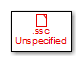6. Double-click the block to open the source file selector dialog box. Select the `my_var_res.ssc` file.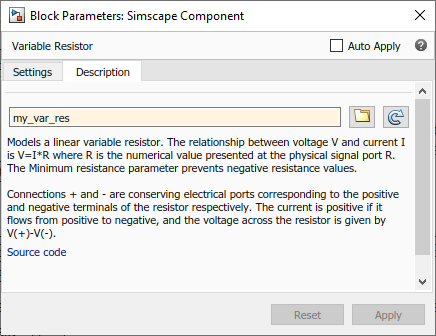7. Close the block dialog box. The block icon gets updated, reflecting the selected source component. It now has two conserving electrical ports, + and –, and a physical signal input port PS.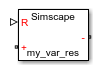8. Double-click the block to open its dialog box. At this point, it has the same block name, description, parameters, and variables, as the Variable Resistor block in the Foundation library.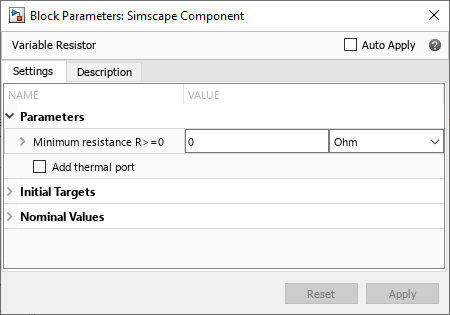9. On the Description tab, click the Source code link to start editing the source code. Change the block name and description:

```component my_var_res % Variable Resistor with Energy Sensor % Variable linear resistor that outputs total electrical energy.```
10. To have the block reflect the changes to the underlying source, on the Description tab, click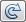. The block dialog box updates accordingly.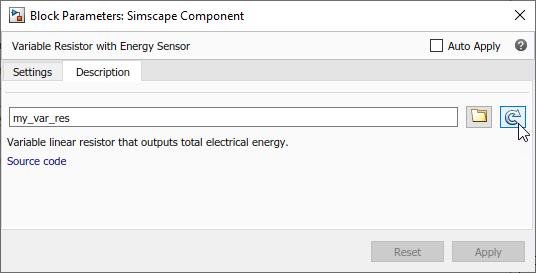11. Declare the output `e` and add the equation calculating total electrical energy. The component source now looks like this:

```component my_var_res % Variable Resistor with Energy Sensor % Variable linear resistor that outputs total electrical energy. inputs R = { 0.0, 'Ohm' }; % PS:left end outputs e = { 0, 'J' }; end nodes p = foundation.electrical.electrical; % +:left n = foundation.electrical.electrical; % -:right end parameters Rmin = { 0, 'Ohm' }; % Minimum resistance R>=0 end variables i = { 0, 'A' }; % Current v = { 0, 'V' }; % Voltage end branches i : p.i -> n.i; end equations assert(Rmin>=0) v == p.v - n.v; if R > Rmin v == i*R; else v == i*Rmin; end e == integ(v*i); end end ```
12. Refresh the block again. Instead of using thebutton on the Description tab, you can right-click the block icon and, from the context menu, select Simscape > Refresh source code.The block icon now has an additional physical signal output port e.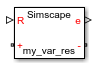13. Connect the block to a simple test rig to verify the correct performance.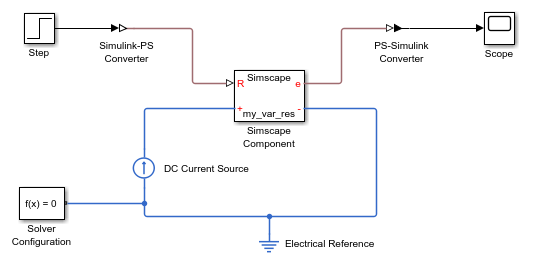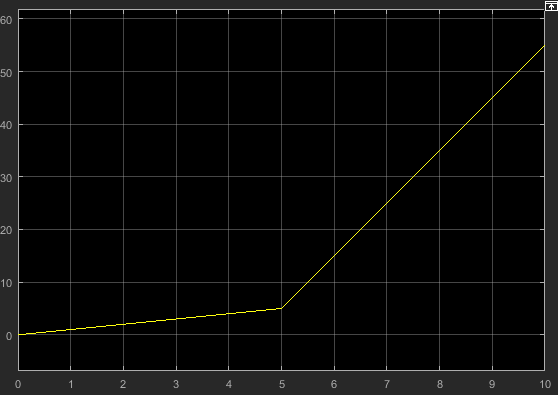Note

There is a limitation that the name of the model cannot be the same as the name of the source file for the Simscape Component block. Therefore, if you save the test rig model, make sure to give it a different name, such as `my_var_res_test`.